# 10 Geometric Art Explorations

Study measurement, area, fractions, and more!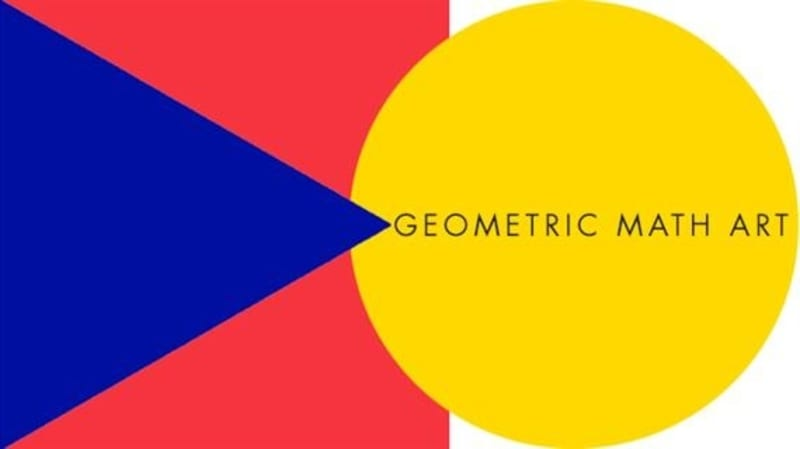In the following activities, students use geometry vocabulary and explore math concepts including: measurement, area and perimeter, fractions and patterns!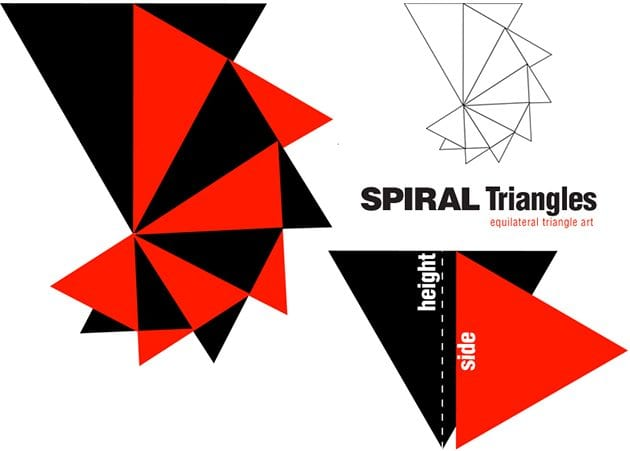## 1. Spiral Triangles

Create a spiral design using equilateral triangles! To create the design, start out with a large triangle. Then, cut out a triangle thats side is the same length of the large triangle’s height. Paste the side of the triangle along the the center of the larger triangle, so it covers half of the large triangle. Keep on creating smaller triangles with sides that match the previous triangle’s height to create a spiral!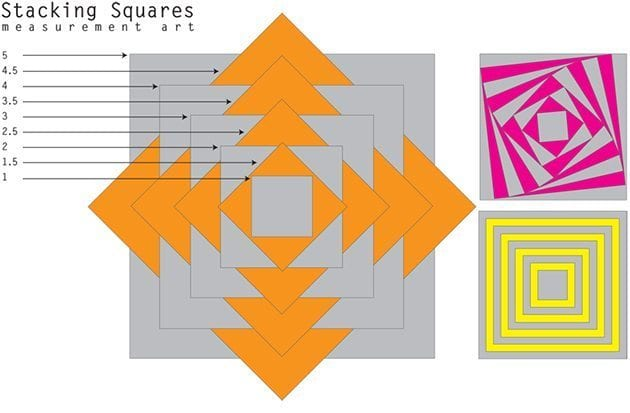## 2. Square Stack

This art activity comes from Lucky To Be In First. Students measure, cut, and paste squares in descending order. Each square is 1/2 an inch smaller than the preceding square.
Note: You could also have children figure out the area and perimeter of each square on a separate sheet of paper.
Example
1. area = 25, perimeter = 20
2. area = 20.25, perimeter = 18
3. area = 16, perimeter = 16
4. area = 12.25, perimeter = 14
5. area = 9, perimeter = 12
6. area = 6.25, perimeter = 10
7. area = 4, perimeter = 8
8. area = 2.25, perimeter = 6
9. area = 1, perimeter = 4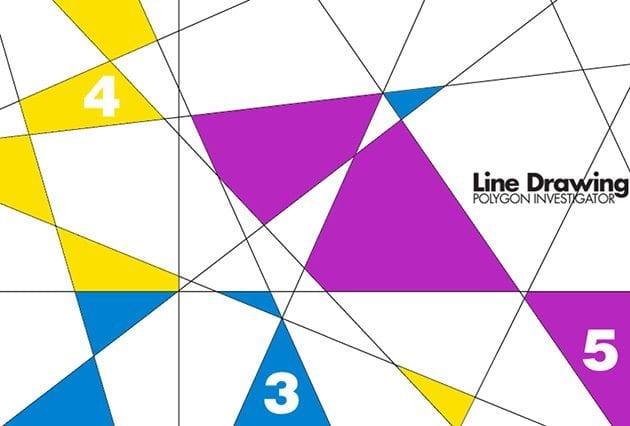## 3. Line Drawing: Polygon Investigator

Integrate math and art by creating a line drawing. Kids use a ruler and draw random lines on paper. Then, they become polygon investigators! They examine their artwork and color in polygons that have the same number of sides.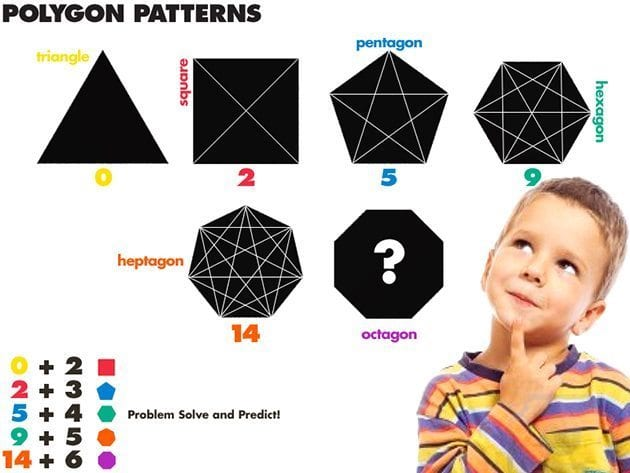## 4. Polygon Patterns

Explore vertices and diagonals while seeking patterns in polygons! Have kids predict the number of diagonals a triangle, square, pentagon, hexagon, heptagon, and octagon have. Split kids up into teams. Then, have them problem solve by drawing diagonals on shape outlines. Make sure kids use different colored markers for each diagonal (so they can count them). Also, have a teammate tally each diagonal drawn. Can they find a pattern?! How many diagonals does a hexadecagon have?!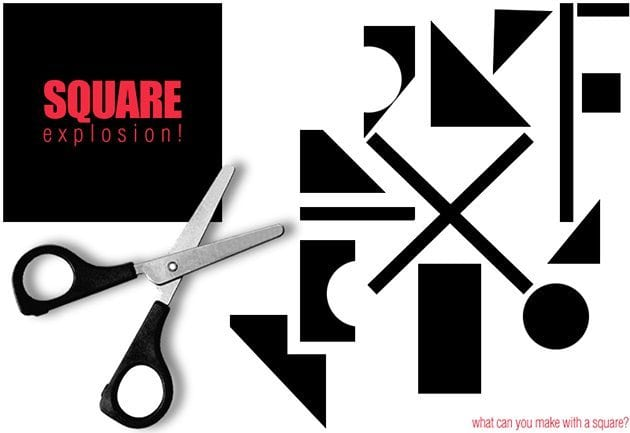## 5. Square Explosion

Give kids scissors and a paper square and see what they can create!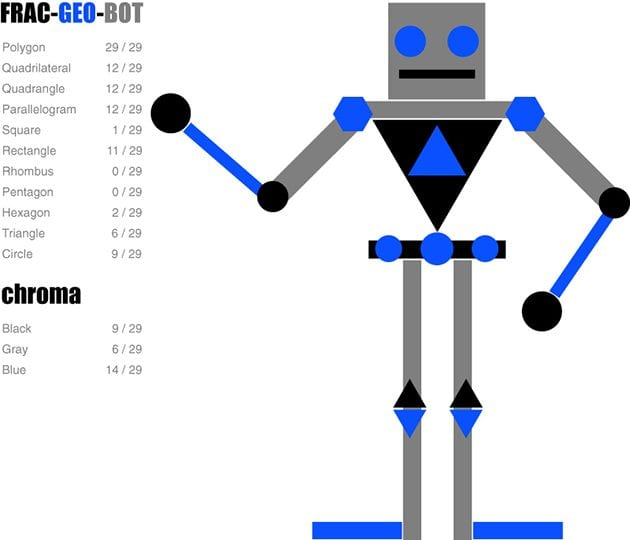## 6. Frac-Geo-Bot

Frac-Geo-Bot is a fun math activity for kids to get creative. They design a robot out of various polygons. Then, they figure out the fraction for each shape and color. Older kids can design more complex bots, like the one above. Younger kids can create simpler bots, using basic shapes.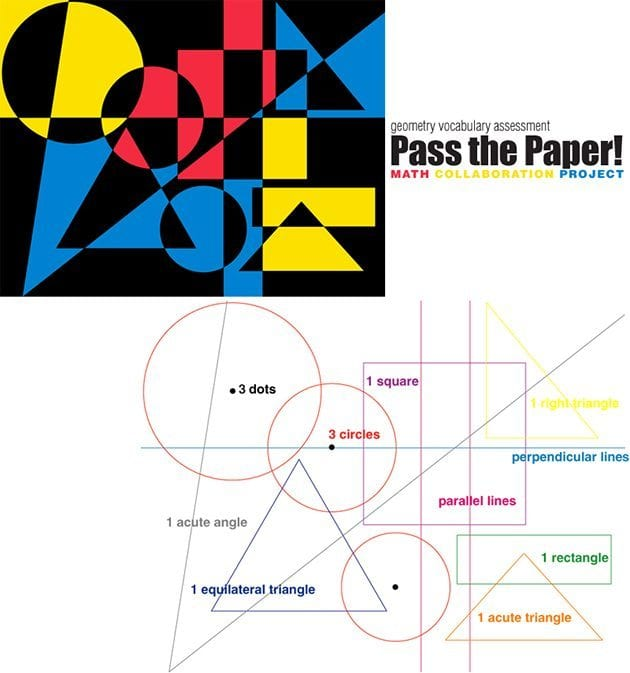## 7. Pass the Paper: Collaboration

This math art collaboration project comes from Incredible Art! Kids show their knowledge of geometry terms by creating art! Each child is given a piece of paper. They are given an instruction (such as make 3 dots). Then, they pass their sheet of paper. Another instruction is given (ex: use a compass to create 3 circles from the 3 dots). Students draw on their peers’ paper. Then, they pass the paper. Papers get passed around until they reach their “owner.” The owner embellishes the line drawing to create a unique piece of art!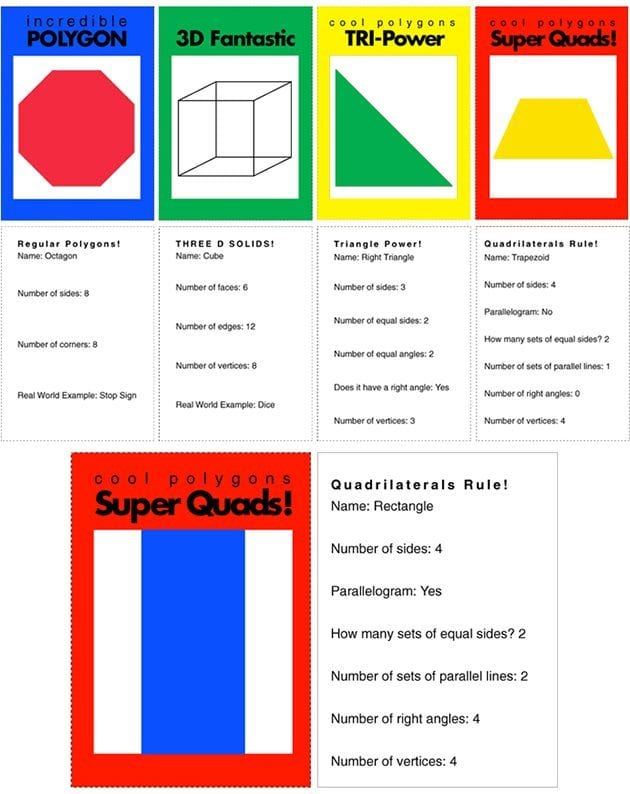Kids draw the shape and fill out the back of the cards. Click here for the printable.

Note: Don’t cut along the center line. The card folds in half, so there is a front and back.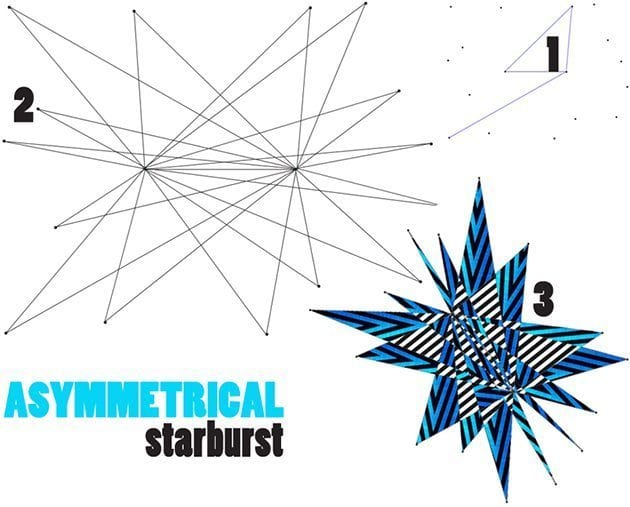## 9. Asymmetrical Starburst

Students explore geometry and art by creating asymmetrical starburst designs!
1. Draw a line segment 3 to 5 inches long in the center of the paper.
2. Make dots around the line segment. (No dots on the horizontal line)
10 dots (5 above, 5 below for younger students / 15-20 dots for older students)
3. Connect the dots! – but, in a pattern. (Connect one end of the line segment to a dot, back to the other end of the line segment) – repeat (end of line segment-dot-other end of line segment) – use a ruler
Note: To simplify this project have children make their horizontal line a different color or add bright colored dots to the end of the line segment. Then, they will be able to see where they draw their lines to.
Math Connection: Visit I Love That Teaching Idea for questions to ask children while they are constructing!

##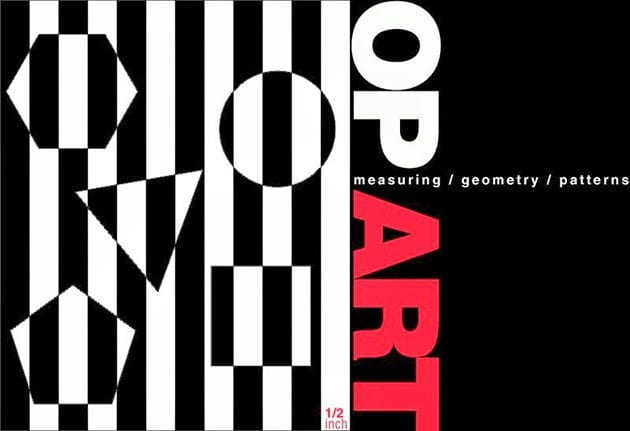10. Mathematical Op Art

Students use their math skills to create op art! First, have students come up with a measurement for their line spacing between their parallel lines. The example has a 1/2 inch gap between each line. After students draw their parallel lines with a ruler, they choose five polygons to include in their composition. They draw their shapes using a ruler. Once their shapes are drawn, they create an AB pattern using two different colored markers, coloring around their shapes. Then, they go back and color their shapes to create an optical illusion! (see example).### Posted by Erin Bittman

Check out my blog eisforexplore.blogspot.com!

+